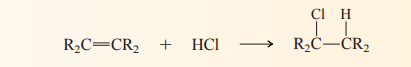# Problem: The overall equation for the addition of HCl to alkenes is:   If the transition state for proton transfer from HCl to the alkene (arrow  5 ) resembles a carbocation and this step is rate-determining, what should be the effect of alkene structure on the rate of the overall reaction? Fastest rate                                    Slowest rateA. H2C=CH2    CH3CH=CHCH3    (CH3)2C=C(CH3)2B. CH3CH=CHCH3    (CH3)2C=C(CH3)2    H2C=CH2C. CH3CH=CHCH3    H2C=CH2    (CH3)2C=C(CH3)2D. (CH3)2C=C(CH3)2    CH3CH=CHCH3    H2C=CH2

###### FREE Expert Solution
87% (358 ratings)
###### Problem Details

The overall equation for the addition of HCl to alkenes is:

If the transition state for proton transfer from HCl to the alkene (arrow  5 ) resembles a carbocation and this step is rate-determining, what should be the effect of alkene structure on the rate of the overall reaction?

Fastest rate                                    Slowest rate

A. H2C=CH2    CH3CH=CHCH3    (CH3)2C=C(CH3)2

B. CH3CH=CHCH3    (CH3)2C=C(CH3)2    H2C=CH2

C. CH3CH=CHCH3    H2C=CH2    (CH3)2C=C(CH3)2

D. (CH3)2C=C(CH3)2    CH3CH=CHCH3    H2C=CH2What scientific concept do you need to know in order to solve this problem?

Our tutors have indicated that to solve this problem you will need to apply the Hydrohalogenation concept. You can view video lessons to learn Hydrohalogenation. Or if you need more Hydrohalogenation practice, you can also practice Hydrohalogenation practice problems.

What is the difficulty of this problem?

Our tutors rated the difficulty ofThe overall equation for the addition of HCl to alkenes is: ...as high difficulty.

What professor is this problem relevant for?

Based on our data, we think this problem is relevant for Professor Zurcher's class at CSU OHIO.

What textbook is this problem found in?

Our data indicates that this problem or a close variation was asked in Organic Chemistry - Carey 8th Edition. You can also practice Organic Chemistry - Carey 8th Edition practice problems.